基于主成分分析的心音特征降维处理研究Principal Component Analysis-Based Heart Sound Features Reduction Research

• 全文下载: PDF(1079KB)    PP.213-219   DOI: 10.12677/JISP.2018.74024
• 下载量: 227  浏览量: 547

In this research, a method based on principal component analysis (PCA) is proposed for using the optimal dimensional features to describe the distribution of heart sound characteristics in different kinds of heart diseases. This study is described in three stages. In stage 1, heart sound signal is collected via 3M-3200 electronic stethoscope and preprocessed based on wavelet transform. In stage 2, the power spectrum density combined with threshold method is proposed to define the cardiac sound 7-dimensional feature. In stage 3, based on principal component selection criteria combined with scatter plot distribution results, the final heart sound is determined to be a 2-dimensional feature representing 96.1% information of 7-dimensional feature. The results of the research on the typical heart diseases indicate that there are obviously differences in the dis-tribution of the heart sound features among different kinds of heart diseases.

1. 引言

2. 心音信号的采集及预处理

2.1. 心音信号的采集

2.2. 心音信号的预处理

3. 心音信号的频域特征提取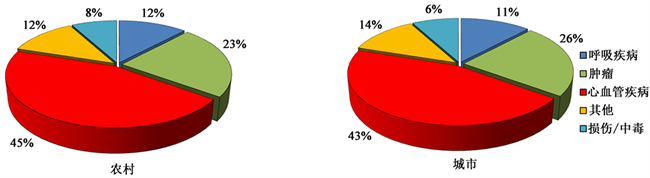Figure 1. Proportion of death among rural and urban residents in China in 2015Figure 2. Collection of heart sounds examples and stethoscope physical chart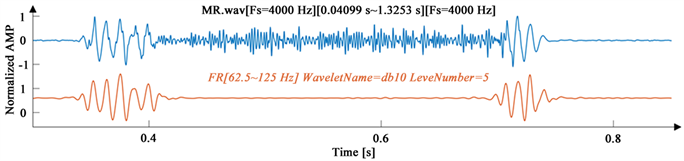Figure 3. An example of noise reduction of mitral regurgitation signal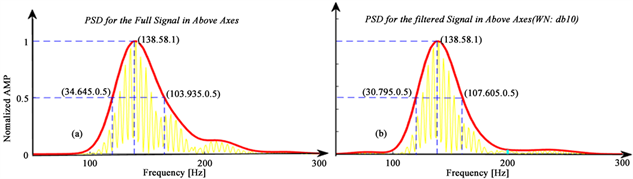Figure 4. Frequency domain feature extraction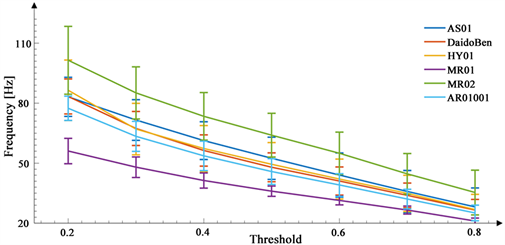Figure 5. Characteristic statistical chart (μ ± σ)(1)

${X}_{{F}_{Sj}}\left[l\right]={\sum }_{n=1}^{{N}_{Sj}}{X}_{{T}_{Sj}}\left[n\right]{\text{e}}^{\left(-j\frac{2\pi }{{N}_{Sj}nl}\right)}l=0,1,2,\cdots ,{N}_{Sj}-1$ (2)

$S{E}_{Sj}\left[k\right]=\frac{1}{2S{L}_{Sj}+1}{\sum }_{l=k-S{L}_{Sj}}^{k+S{L}_{Sj}}|{E}_{Sj}\left[l\right]|,k=S{L}_{Sj},\cdots ,{N}_{Sj}-1-S{L}_{Sj},j=1,2,3$ (3)

4. 降维处理研究

4.1. 主成分分析的简介

1) 对原始数据进行标准化处理(消除量纲影响)

${\chi }_{ij}^{*}=\frac{{\chi }_{ij}-{\stackrel{¯}{\chi }}_{j}}{\sqrt{Var\left({\chi }_{j}\right)}}$ (4)

$j=1,2,3,\cdots ,m$

2) 计算数据的协方差矩阵

$X=\left({s}_{ij}\right)$ (5)

3) 求出矩阵的特征值及相应的正交化单位特征向量

4) 计算各特征值的贡献率及累计贡献率

$\frac{{\lambda }_{i}}{{\sum }_{k=1}^{m}{\lambda }_{k}}$ (6)

$\frac{{\sum }_{k=1}^{i}{\lambda }_{k}}{{\sum }_{k=1}^{m}{\lambda }_{k}}$ (7)

4.2. 特征降维处理结果Table 1. Principal component analysisTable 2. Eigenvalue and its cumulative contribution rate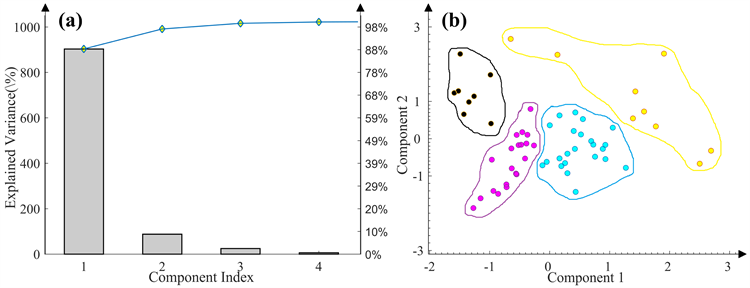Figure 6. Principal component analysis results

5. 结论

  《中国心血管病报告2017》概要[J]. 中国循环杂志, 2018(1): 1-8.  罗玉光, 心脏听诊的基础知识——怎样听心音[J]. 人民军医, 1964(7): 41-44.  https://www.littmann.com  陈新华, 成谢锋. 一种改进型综合去噪算法在心音信号预处理上的研究[J]. 南京邮电大学学报(自然科学版), 2010, 30(6): 96-100.  单正娅. 基于人工神经网络及小波分析的心音诊断系统的研究[D]: [硕士学位论文]. 无锡: 江苏大学, 2006.  Sun, S.P., et al. (2014) Segmentation-Based Heart Sound Feature Extraction Combined with Classifier Models for a VSD Diagnosis System. Expert Systems with Applications, 41, 1769-1780. https://doi.org/10.1016/j.eswa.2013.08.076  刘立汉, 王海滨, 王燕, 陶婷, 魏秀波. 基于改进的希尔伯特–黄变换的心音信号特征分析[J]. 西华大学学报(自然科学版), 2010, 29(6): 14-18.  胡玉良, 王海滨, 陈健, 等. 心音在时频两域中解析方法的研究[J]. 西华大学学报(自然科学版), 2009(5): 5-8, 26.  Joy, R., Acharya, U.R., Mandana, K.M., Ray, A.K. and Chakraborty, C. (2012) Expert Systems with Applications Application of Principal Component Analysis to ECG Signals for Automated Diagnosis of Cardiac Health. Expert Systems with Applications, 39, 11792-11800. https://doi.org/10.1016/j.eswa.2012.04.072  Giri, D., Acharya, U.R., Martis, R.J., Sree, S.V., Lim, T.-C., Ahamed, T. and Suri, J.S. (2013) Automated Diagnosis of Coronary Artery Disease Affected Patients Using LDA, PCA, ICA and Discrete Wavelet Transform. Knowledge-Based Systems, 37, 274-282. https://doi.org/10.1016/j.knosys.2012.08.011  Lee, J. and Jun, C.-H. (2013) PCA-Based High-Dimensional Noisy Data Clustering via Control of Decision Errors. Knowledge-Based Systems, 37, 338-345. https://doi.org/10.1016/j.knosys.2012.08.013  Shilaskar, S. and Ghatol, A. (2013) Feature Selection for Medical Diagnosis: Evaluation for Cardiovascular Diseases. Expert Systems with Applications, 40, 4146-4153. https://doi.org/10.1016/j.eswa.2013.01.032  Zhu, B., Ding, Y. and Hao, K. (2014) Multiclass Maximum Margin Clustering via Immune Evolutionary Algorithm for Automatic Diagnosis of Electrocardiogram Arrhythmias. Applied Mathematics and Compu-tation, 227, 428-436. https://doi.org/10.1016/j.amc.2013.11.028